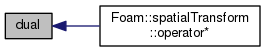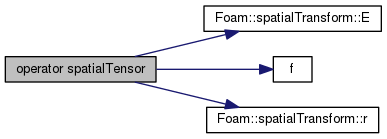The OpenFOAM Foundation
spatialTransform::dual Class Reference

Wrapper-class to provide dual functions and operators. More...

## Public Member Functions

dual (const spatialTransform &X)
Construct from a spatialTransform. More...

operator spatialTensor () const
Return dual transformation tensor ^B{X^*}_A. More...

spatialVector operator & (const spatialVector &f) const
Transform dual f: ^B{X^*}_A & f. More...

## Detailed Description

Wrapper-class to provide dual functions and operators.

Definition at line 108 of file spatialTransform.H.

## ◆ dual()

 dual ( const spatialTransform & X )
inline

Construct from a spatialTransform.

Definition at line 69 of file spatialTransformI.H.

Referenced by spatialTransform::operator*().

Here is the caller graph for this function:## ◆ operator spatialTensor()

 operator spatialTensor ( ) const
inline

Return dual transformation tensor ^B{X^*}_A.

Definition at line 204 of file spatialTransformI.H.

References spatialTransform::E(), f(), spatialTransform::r(), and Foam::Zero.

Here is the call graph for this function:## ◆ operator &()

 spatialVector operator& ( const spatialVector & f ) const
inline

Transform dual f: ^B{X^*}_A & f.

X^* . f = (E . fw - r ^ fl, E . fl)

The documentation for this class was generated from the following files: